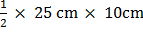### Sample Problem

In triangle ABC, the height AD = 10 cm, the length of CD is half the length of AD, and the length of BD is twice the length of AD. What is the area of Triangle ABC?The area of Triangle ABC is cm2.

#### Solution

DC = AD ÷ 2 = 10 cm ÷ 2 = 5 cm

BD = 2 × AD = 2 × 10 cm = 20 cm

BC = BD + DC = 20 cm + 5 cm = 25 cm.=== 125 cm2

The area of Triangle ABC is 125 cm2